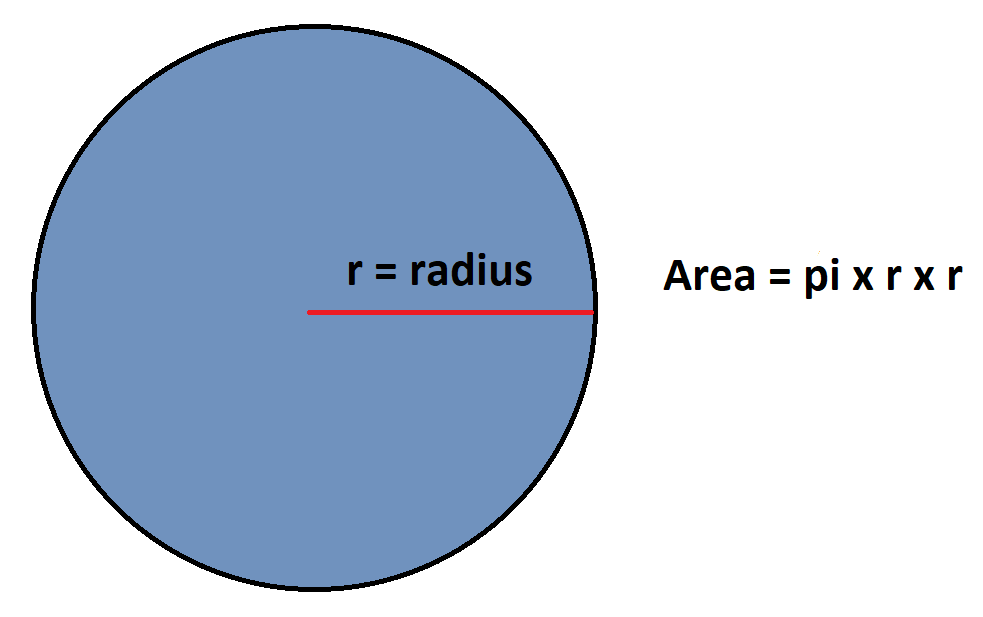Parenting

# The Pizza Equation

358 views

 1 Introduction 2 Area of circle 3 The Pizza Equation 4 Summary 5 Frequently Asked Questions (FAQs)

30 September 2020

## Introduction

In this blog, Gauri, a proud Cuemath student, explains a simple real-life problem and how we can make better decisions if we involve some math in our lives.

## Area of circle

The following Pizza equation in this blog operates on a fundamental yet straightforward math concept, which is finding surface area. We will use the area of the circle in this blog to understand the pizza equation better.

Area of the circle comes very handy in math and is frequently applied in real-life situations. Whether you have to find the area of a cricket ground( considering it a circle) or find out how much grass can horse eat while tied to a post with a rope, you can use the area of circle to reach the answer.Related Articles
GIVE YOUR CHILD THE CUEMATH EDGE
Access Personalised Math learning through interactive worksheets, gamified concepts and grade-wise courses